Courses
Courses for Kids
Free study material
Free LIVE classes
More

# Learn about 2D Shapes: Definition, Formulae, and Examples with Pictures## Area of 2-Dimensional Shapes

In Geometry, a shape is defined as a figure that is enclosed by a boundary. The border of the shapes is formed by lines, points, and curves. Two-dimensional (2D) shapes are bordered by straight lines. The 2D shapes have only length and breadth and they are popularly known as polygons and flat shapes. These include squares, rectangles, circles, rhombuses, and triangles. The area of a 2D shape is the region enclosed within the boundary which is measured in square units. Now, we will discuss more details about basic 2-dimensional shapes and their Areas.

## Area of a Rectangle

The area of a rectangle in a two-dimensional region is the area covered by the rectangle. A rectangle is a two-dimensional form with four sides and four vertices. All the four angles in a rectangle are 90 degrees. The opposite sides of the rectangle are equal and parallel to one another.

Formula: Area of a rectangle = Length (L) x Breadth (W) (or width)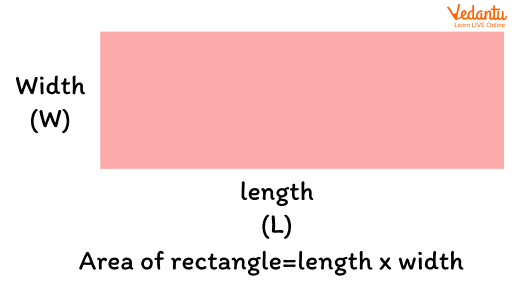The shaded region represents the area of a rectangle with length and width

Example: Find the area of a rectangle with a length of 4 metres and a width of 2 metres.

Ans: Given length L = 4 m and width (W) = 2m

Area of the given rectangle = 4 x 2 = 8 sq.m

## Area of a Square

A Square is a 2-Dimensional shape just like a rectangle. The only difference between a square and a rectangle is that in a rectangle only opposite sides are equal whereas in a square shape, all sides are equal. We can say a square is a rectangle with all of its sides being the same length.

Formula: Area of a square = side x side = (side)2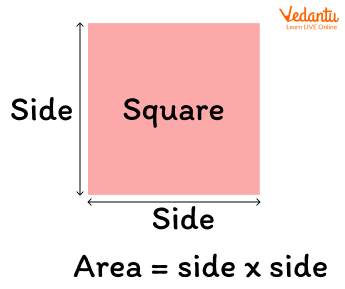The shaded region represents the area of a square

Example: Find the area of a square whose side measures 3 cm.

Ans: Given the side of a square = 3cm

So, the area of the square = 3 x 3 = 9 sq.cm.

## Area of a Circle

A circle is a closed curve with an exterior line that is equidistant from the centre. The radius of the circle is the fixed distance from the centre point to the boundary of the circle. Many examples of the circle can be found in everyday life, such as a wheel, pizzas, a round ground, and so on.

Formula: Area of circle with radius ‘r’ is given by the formula A = πr2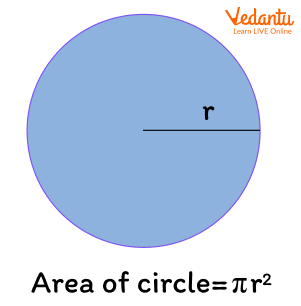Example: Find the area of a circle whose radius is 5 metres.

Ans: Given, radius of the circle r = 5m

Therefore, area of the circle = π(5)2= π(25) = 25 square units

## Area of a Triangle

A triangle is one of the most fundamental 2-Dimensional shapes with three sides and three vertices. The area of the triangle is the region occupied inside the boundary of the triangle.

Formula: Area of a triangle with base ‘b’ and height ‘h’ = 1/2 base x height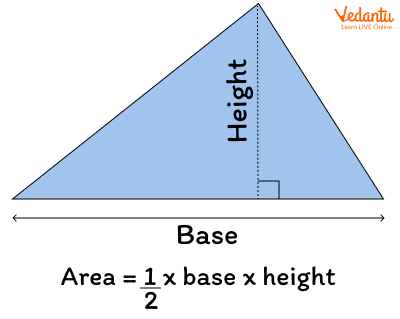The shaded region represents the area of a triangle with base (b) and height (h).

Example: Find the area of the triangle with height 4m and base 3m.

Ans: Given, the height of the triangle (h) = 4m

The base of the triangle (b) = 3m

Area of the triangle = $\frac{1}{2}$ x 4 x 3 = 6 sq.m

## Area of an Equilateral Triangle

An equilateral triangle is a type of triangle in which all sides are equal in length.

Formula: Area of an equilateral triangle = $\frac{\sqrt{3}}{4}$ x side2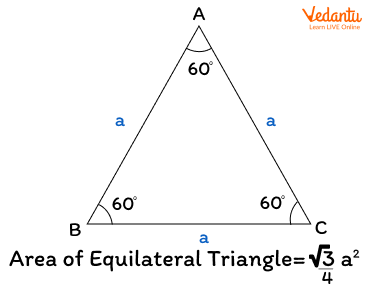The shaded region in the above image shows the area of an equilateral triangle with side ‘a’.

Example: Find the area of an equilateral triangle with side length equal to 2m.

Ans: Given the side of an equilateral triangle is 2m.

So, the area of the given equilateral triangle is = $\frac{\sqrt{3}}{4}$ x (2)2 = $\sqrt{3}$ sq. m

## Area of a Parallelogram

A parallelogram is a quadrilateral, in which opposite sides are equal in length and parallel but the angles are not equal to 90 degrees.

Formula: The area of a parallelogram with base ‘b’ and height ‘h’ is

A = base (b) x height (h)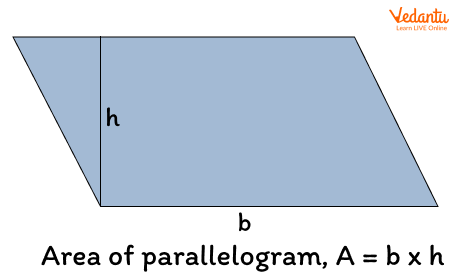The shaded region shows the area of a parallelogram

Example: Find the area of a parallelogram with a base length of 9m and height of 3m.

Ans: Given, parallelogram has base length b = 9m and height h = 3m.

So, the area of the given parallelogram is A = 3 x 9 = 27 sq.m

## Areas of 2D Shapes Chart

The below chart shows various two-dimensional shapes with their corresponding formulas which can be kept handy to refer to in future.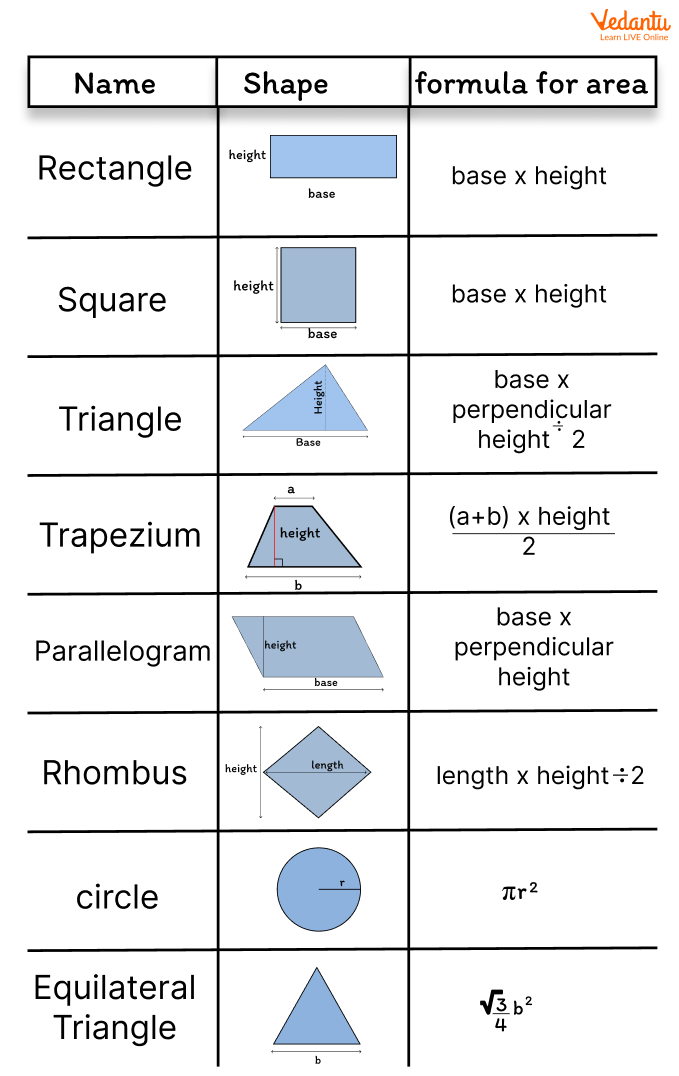The above table shows 2D shapes and their corresponding areas.

## Conclusion

In Mathematics, 2D shapes are planar figures that can be drawn on a flat surface or a sheet of paper. Two-dimensional shapes have sides and corners, while others have curved boundaries. The area of a 2-D shape is the region enclosed within the boundary of the shape. Learning 2D shapes and their areas is fun and helps the kids to improve their geometry skills.

Last updated date: 15th Sep 2023
Total views: 132.6k
Views today: 4.32k

## FAQs on Learn about 2D Shapes: Definition, Formulae, and Examples with Pictures

1. What are 2D shapes?

The term 2D refers to two-dimensional. Two-dimensional shapes are plane (flat) shapes which contain only two dimensions: length and width. Squares, rectangles, circles, triangles, and other shapes are among them. The shape of a 2D is defined by the number of sides. For example, a triangle is a three-sided planar shape having three corners. A rectangle is a four-sided and four-cornered shape.

2. What is the area of 2D shapes?

The size of the region enclosed within any 2D shape is called its area. It is the space occupied by the object on the surface. The number of unit squares that cover a figure's surface is known as its area. Square units such as square centimetres, square feet, square inches, and so on are used to measure area.